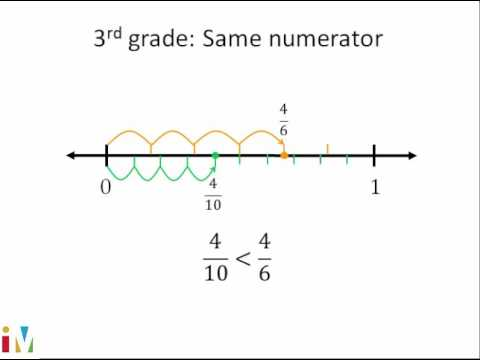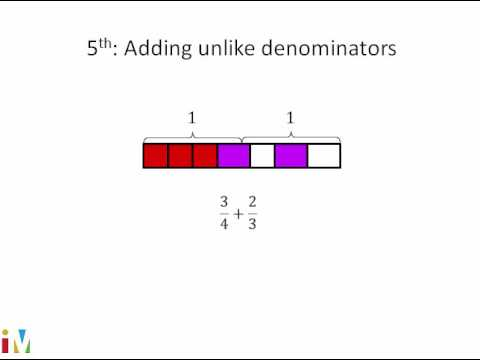Video

# Finding common denominators (Full video)

Description: A short lesson on how to rewrite 2/8 and 5/6 to have the same denominator. We're asked to rewrite the following two fractions as fractions with a least common denominator. So a least common denominator for two fractions is really just going to be the least common multiple of both of these denominators over here. And the value of doing that is then if you can make these a common denominator, then you can add the two fractions.

### Other videos you might be interested in### Comparing Fractions

#### Illustrative Mathematics